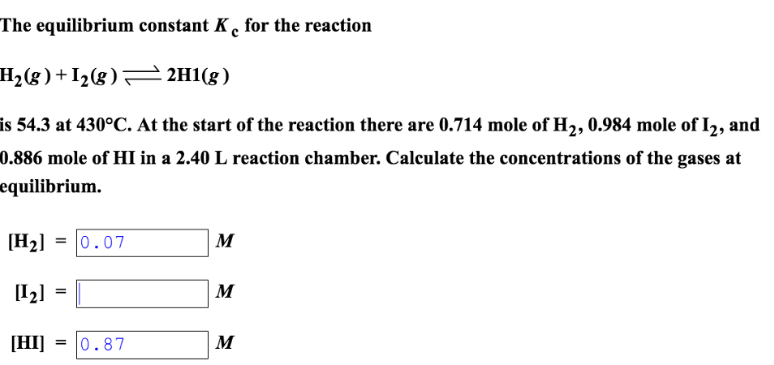# Problem: The equilibrium constant Kc for the reactionH2(g) + I2(g) ⇌ 2HI(g)is 54.3 at 430 C. At the start of the reaction, there are 0.714 mole of H_2, 0.984 mole of I2, and 0.886 mole of HI in a 2.40 L reaction chamber. Calculate the concentrations of the gases at equilibrium.

###### FREE Expert Solution
89% (102 ratings)###### Problem Details

The equilibrium constant Kc for the reaction

H2(g) + I2(g) ⇌ 2HI(g)

is 54.3 at 430 C. At the start of the reaction, there are 0.714 mole of H_2, 0.984 mole of I2, and 0.886 mole of HI in a 2.40 L reaction chamber. Calculate the concentrations of the gases at equilibrium.